## 7805 voltage regulator for your design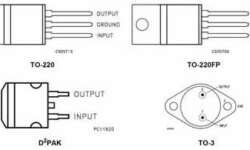This is the most common voltage regulator that is still used in embedded designs. The LM7805 voltage regulator is a linear regulator made by several manufacturers like Fairchild or ST Microelectronics. They can come in several types of packages. For output current up to 1A, there may be two types of packages: TO-220 (vertical) and D-PAK (horizontal). With a proper heat sink, these LM78xx types can handle even more than 1A current. They also have Thermal overload protection, Short circuit protection.

## Understanding Timing diagrams of digital systemsTiming diagrams are the main key in understanding digital systems. Timing diagrams explain digital circuitry functioning during time flow. Timing diagrams help to understand how digital circuits or sub-circuits should work or fit into a larger circuit system. So learning how to read Timing diagrams may increase your work with digital systems and integrate them. Bellow is a list o most commonly used timing diagram fragments: Low level to supply voltage:

## Control motor PWM schematicIn general, there are two ways to control DC motor speed: by varying supply voltage and pulse width modulation (PWM). The first control method is not convenient, especially in digital systems. It requires analog circuitry, and so on. The second motor speed control method is very convenient for digital systems because all control is made using only digital signals. As you already know, PWM (Pulse Width Modulation) is all about switching speed and pulse width (duty cycle). Duty cycle is the ratio of signal time ON/T. T is the period of the signal. In the above diagram, you see two signals. The first duty cycle is about t1/T=1/3, and another’s duty cycle would be about t2/T=2/3. And notice the period of signals are the same.

## Power sources for AVR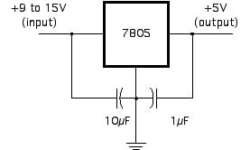Power sources for AVR microcontrollers are a crucial part. Every circuit has to be powered from some source like a battery or AC adapter 110V/220V. Using batteries is a more convenient way to power the microcontroller projects as the circuits are simpler and constructed devices become portable. There are many types of batteries in shapes and sizes or capacities. So when choosing a battery you should consider many factors: Capacity is a critical parameter measured in mA/h. This parameter defines how long your microcontroller project will be working before recharging or replacing batteries. The rule is simple – as bigger battery capacity, as long your circuit will be working. Still, on the other hand, your project may become more expensive or even heavier because of bigger batteries. The second parameter is the battery Voltage. If the battery’s voltage is too small for your circuit, you’ll have to connect several batteries in series. Another is the Expiry date. You don’t want your battery energy leakage or become obsolete because of this. Working temperature. If your project is working in more extreme temperatures – heat or cold, you should consider this. And the last parameter would be chape, size, and weight. If…

## Consider PWM signal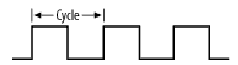When using a microcontroller and want to drive motor control or controlled intensity, you can use DAC to generate an analog output voltage. But there is an easier way of doing this. You can use a digital output to reach the same results. This technique is known as PWM -Pulse Width Modulation. In this picture, you can see a 50% duty cycle square waveform. The width of ‘0’ is equal to the ‘1’ level. This means if the signal amplitude is 5V, then the average voltage over one cycle is 2.5V. It is as though of hawing constant 2.5V voltage.

## K-Type Thermocouples reviewed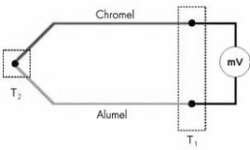A thermocouple is a sensor that generates an electrical potential related to the temperature. The sensor’s operating principle is based on the fact that any electrical junction between two different metals generates an electrical potential that depends on the temperature and the metals used. The principle applies equally well if three metals are used. In that case, there are two junctions in series and the net potential results from the series addition of the two individual potentials. For example, if a copper iron junction is in series with an iron-tin junction, the net potential is the same as for a copper-tin junction. However, that is only true if both junctions are at the same temperature. The K-Type thermocouple is usually made of Chromel (+) and Alumel(-). The voltage generated from this sensor is 4mV/100°C. The max temperature that can thermocouple withstand is 1000°C without any damage.

## Impulse signal distortion in the transmission line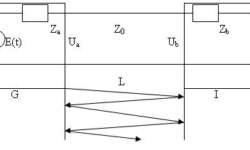Today most electronic equipment consists of signal generators and processing units. These units are connected with transmission lines. These lines have a big influence on signal distortions. On these lines depends transmission lines stability. Let’s see how transmission lines affect transmitted signals. (G- signal generator; Za– output impedance; I- signal receiver Zb– input impedance; L- transmission line length; Z0– Line impedance. When line is tuned and without losses then input voltage: Ub(t) = ZbE(t-t)/(Za + Zb) E(t)- generators signal amplitude; t- signal delay in line. t =l/v ; l- line lenght; v- signal speed. If the line is not tuned up, then there are distortions in line because of reflections inline: If signal E(t) is step function: Then signal in line exit in discrete time moments will be: U(0) = p; U(t) = p*(1+pb) U(2t) = p*(1+pb+pa*pb) U(3t) = p*(1+pb+pa*pb+pa*pb^2); U(4t) = p*(1+pb+pa*pb+pa*pb^2+pa^2*pb^2); U(5t) = p*(1+pb+pa*pb+pa*pb^2+pa^2*pb^2+pa^2*pb^3) â€¦ where Depending on reflectance coefficients and their signs distortions can differentiate or integrate: A real model using MathCAD was implemented to see how the signal looks on exit depending on parameters. Below you see used algorithm structure used in modeling: Part 2: One of results using trapezoid signal: In exit we get distorted…

## Filter output dependency on tolerance of elements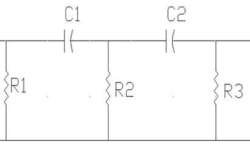Sometimes we need to project a simple filter with particular characteristics. We usually take calculations with ideal parameters and don’t look at tolerances of them. Let us see how simple filter output depends on its elements tolerances. For this let’s choose simple filter circuit: We are going to calculate the filter response characteristics. The bandpass frequency is taken on 0.707 level of response. We will see how this frequency depends on the electronic element tolerances. I will model elements with tolerances ±10%.

## Z axis accelerometer MMA1220D from Freescale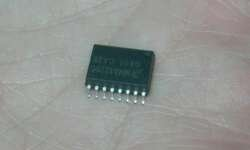MMA1220D is Z-axis MEMS accelerometer -8g – +8g. This sensor has a capacitive sensor. The output signal is passed through a 4 poles low pass filter. It also has an internal self-test capability. The main features:Integral signal conditioning;Linear output;Ratiometric performance;4th order Bessel Filter to preserve pulse shape integrity;Calibrated self-test;Detection of low voltage also clock monitor and EPROM Parity check status;Can survive big shocks. Where can it be used? It can be the perfect tool for Vibration monitoring, control, bearing monitor, PC HDD protection, mouse and joystick, virtual reality, sports diagnostics.The datasheet can be found here:https://www.freescale.comI decided to run a sample test for this sensor. For this, I just made a simple board where I soldered this sensor with the SOIC16 package. According to the datasheet, I put an RC filter on sensor output to minimize clock noise. There is a schematic: And traced PCB: I used SMD parts as it will be easier to glue another side of the board to any surface in the future. First test drive on oscilloscope: Later I will do some tests by connecting this sensor to MCU.Bellow, I attached the Protel library of the MMA1220D sensor and my project files. It might be you…

## Physiological system modeling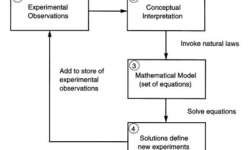Most physical and chemical experiments can be described with a finite number of rules. In physiological processes, everything can be tied to the laws of nature. A good model can be a guideline for new experiments. Usually, the model and experiment have an iterative connection. To understand how to investigate any material, there is a model needed (block 1). First, we need an abstract understanding of what we are investigating (block 2). Abstracts are transformed into mathematical models (block 3). There we can use formulas and solve them using the computer. The results you get shows, what experiments have to be done, and what to expect from them. This loop system is resistive to irregularities in the mathematical model. In other words, this is the purifier of experiments and models.